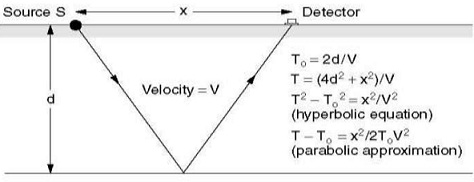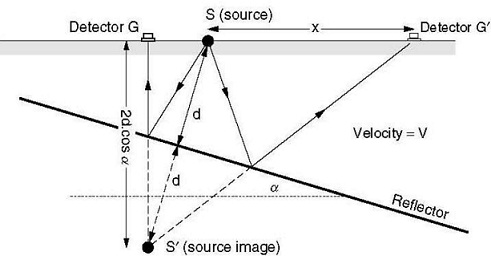#### Seismic Reflection, Physics tutorial

Introduction:

On applied geophysics the seismic reflection method absorbs more than 90% of the money used on world-wide. Several surveys are focus at defining oil bearing structures at depths of thousands of meters using hundreds or even thousands of detectors. However, few reflection works is completed by small field crews probing to depths of, at most, a few hundred meters. The devices used in these surveys were originally very easy but may now have as much in-built processing power as the massive processing laboratories of 20 years ago. A Field operator requires having some understanding of the theory in front the options available.

Reflection Theory:

As previously used Ray-path diagrams offers useful insights into the timing of reflection events but supplies no indication of amplitudes.

Reflection Coefficients And Acoustic Impedances :

I denotes   the acoustic impedance of a rock, is equal to its density multiplied by the seismic P-wave velocity. If a seismic wave front strikes a planar interface between two rock layers with impedances I1  and  I2  at right angles (normal incidence), the amplitude of the reflected wave, whereas a percentage of the amplitude of the incident wave (the reflection coefficient,  RC) is given by:

RC = (I2 - I1) / (I2 + I1)

If the coefficient is negative, I1 is greater than I2, and the wave is reflected with phase returned, that is. A negative pulse will be reversed where a positive pulse was transmitted and vice versa. The amount of energy reflected reduced firstly and then increases as the angle of incidence increases. If the velocity is more in the second medium than in the first, there is no transmitted wave and ultimately total reflection. Thus, most small-scale surveys use waves reflected at nearly normal incidence.

Normal Move-Out:

In survey work the true normal-incidence ray cannot be used, since a detector at the shot point would possibly be destroyed and would surely be set into such violent oscillation that the whole record would be unusable.Fig: Derivation of the Normal move-out Equation for a horizontal Reflector (T is the normal incidence time)

Therefore Geophones are offset from geometric corrections and sources must be made to travel times. Figure given below shows reflection from a horizontal interface, depth d, to a geophone at a distance x from the source. The accurate hyperbolic equation linking the travel time T and the normal incidence time to is established by application of the Pythagoras theorem. For little offsets, the complete equation can be replaced by the parabolic approximation, which gives the normal move out (NMO), T - to, directly as a function of velocity, reflection time and offset. T - To = x2/2V 2To Since  V  usually grows with depth and  To always does, NMO reduced that is NMO curves flatten with depth. On many multi-channel records Curved alignments of reflection events can be seen. Curvature is the most reliable way of distinctive shallow reflections from refractions.

Dix Velocity:

When there is several different layers above a reflector, the NMO equation will give the 'root-mean-square' (RMS) velocity defined as: V2RMS = (V21 t1 + V22 t2 · · ·+· · · V2n tn)/Tn where, velocity Vn, tn is the transit time by the nth layer and Tn is the total transit time to the base of the nth layer. Interval velocities can be calculated from RMS velocities using the Dix formula:

V2DIX = (V2n-1Tn-1 - V2n Tn)/(Tn-1 - Tn)

Refractions were generated by the earlier events were produced. Reminds that on Channels 11 and 12 the strong refracted wave completely overloads the reflection. The variable area representation used is popular for reflection work since it emphasizes trace-to-trace correlations, though some information is lost where records overlap.   the top and bottom of the  nth layer denoted by the  subscripts n - 1 and n  RMS  velocities are generally to some extent higher than true average velocities, since squaring the high velocities raised their influence on the average. Significant exception can arise if RMS velocities are used directly to make depth estimates but these are commonly less than the errors introduced by the use of the NMO equation to estimate velocity using reflections from interfaces that may well not  horizontal. Dix alteration may not help very much in these cases.

Effect of Dip:

If the source is located at the centre of the geophone scattered, the curves achieved over horizontal interfaces will be symmetrical about the point of source. If, however, the reflector has a uniform dip α, the reduction in travel path on the up-dip side of the shot compensates to some extent for the offset, and some travel times will be less than the normal-incidence time. The minimum time 2d  ·  cos(α)/V  is recorded at a distance 2d  ·sin(α) from the shot, on the up-dip side. The reflected ray rises vertically to this point, about which the move-out curve is symmetrical. Dip effects in shallow reflection surveys are detectable only for very large dips or very long spreads.Fig: Effect of Dip on a Single-fold Record

Rays are reflected from the sinking interface as if originate from the image point S_ at depth 2d · cos α below the surface, where d is the perpendicular distance from the shot-point to the interface. The normal incidence journey time is 2d/V but the shortest travel time is for the ray which is vertical after reflection. An identical move-out hyperbola would be produced by a shot at point G and a horizontal interface at depth d · cos α.Fig: Paths for Multiple Reflections: (a) Simple Multiple (b) Peg-leg (c) Intra-formational Multiple

Multiple Reflections:

A wave reflected upwards with high amplitude from a subsurface interface can be reflected down again from the ground surface and then back from the same interface. This is an easy manifold. Two strong reflectors can originate peg-leg and intraformational multiples. Multiples are difficult to identify with certainty on single traces. They can sometimes be considering on multi-channel records because they have move-outs suitable to shallow reflectors and simple time relationships with their primaries.

Tutorsglobe: A way to secure high grade in your curriculum (Online Tutoring)